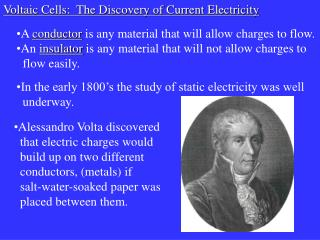DownloadDownload PresentationA conductor is any material that will allow charges to flow. An insulator is any material that will not allow charge

# A conductor is any material that will allow charges to flow. An insulator is any material that will not allow charge

Download Presentation## A conductor is any material that will allow charges to flow. An insulator is any material that will not allow charge

- - - - - - - - - - - - - - - - - - - - - - - - - - - E N D - - - - - - - - - - - - - - - - - - - - - - - - - - -
##### Presentation Transcript

1. Voltaic Cells: The Discovery of Current Electricity • Alessandro Volta discovered • that electric charges would • build up on two different • conductors, (metals) if • salt-water-soaked paper was • placed between them. • A conductor is any material that will allow charges to flow. • An insulator is any material that will not allow charges to • flow easily. • In the early 1800’s the study of static electricity was well • underway.

2. Negative charge build-up Zinc Salt-water- soaked paper Positive charge build-up Copper • The metal plates are called electrodes. • The water-soaked paper is called the electrolyte.

3. Volta named the positive end of the • pile the cathode, and the negative end • the anode. • If the anode and cathode are • connected by another conductor, the • charges will flow from one to the • other. • Volta found that by adding more layers, he could increase • the amount of charge that would build up. • This is called a Voltaic Pile; a very • early version of the battery.

4. Volta, and many other scientists at the time, (Ben Franklin for example) assumed that the positive charge would flow out of the cell. • Conventional current, (the • flow of positive charge) • was widely accepted until • the discovery of the electron • several decades later.

5. Charge • Charge is measured in groups called coulombs. • A coulomb is a group of 6.25x1018 charges. • The amount of charge in an object can be calculated • as follows: where, Q = amount of charge, (coulombs, “C”) N = number of charges e = elementary charge 1.6 x 10-19 C

6. Voltage • Voltage is a measure of the energy carried a coulomb • of charge. Where V = voltage, (V) E = energy, (J) Q = amount of charge, (coulombs, “C”) • Potential difference refers to the change in voltage of • charges in two different parts of a circuit.

7. Example #1 Find the potential difference of a dry cell if it does 0.35 J of work moving 1.56 x 1016 charges.

8. Electric Current Current is the rate at which charge flows in a current. It can be found by measuring the amount of charge that passes a given point in a circuit per second. where I = current, (amps, “A”) Q = amount of charge, (C ) t = time, (s)

9. Example #2 It takes a current of 1.0x101 A 30.0 s to boil a kettle of water. If this kettle requires 360 000 J of thermal energy, what is the potential difference across the kettle?

10. Homework pg. 441 #1-8, 10 pg. 445 #1-6